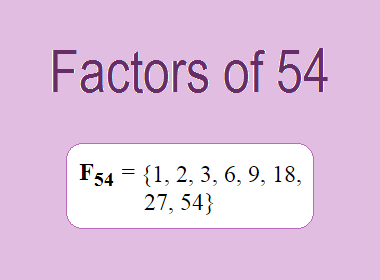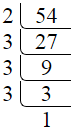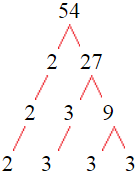# Factors of 54The factors of 54 are 1, 2, 3, 6, 9, 18, 27, and 54 i.e. F54 = {1, 2, 3, 6, 9, 18, 27, 54}. The factors of 54 are all the numbers that can divide 54 without leaving a remainder.

We can check if these numbers are factors of 54 by dividing 54 by each of them. If the result is a whole number, then the number is a factor of 54. Let's do this for each of the numbers listed above:

·        1 is a factor of 54 because 54 divided by 1 is 54.

·        2 is a factor of 54 because 54 divided by 2 is 27.

·        3 is a factor of 54 because 54 divided by 3 is 18.

·        6 is a factor of 54 because 54 divided by 6 is 9.

·        9 is a factor of 54 because 54 divided by 9 is 6.

·        18 is a factor of 54 because 54 divided by 18 is 3.

·        27 is a factor of 54 because 54 divided by 27 is 2.

·        54 is a factor of 54 because 54 divided by 54 is 1.

## How to Find Factors of 54?

1 and the number itself are the factors of every number. So, 1 and 54 are two factors of 54. To find the other factors of 54, we can start by dividing 54 by the numbers between 1 and 54. If we divide 54 by 2, we get a remainder of 0. Therefore, 2 is a factor of 54. If we divide 54 by 3, we get a remainder of 0. Therefore, 3 is also a factor of 54.

Next, we can check if 4 is a factor of 54. If we divide 54 by 4, we get a remainder of 2. Therefore, 4 is not a factor of 54. We can continue this process for all the possible factors of 54.

Through this process, we can find that the factors of 54 are 1, 2, 3, 6, 9, 18, 27, and 54. These are the only numbers that can divide 54 without leaving a remainder.

********************

********************

## Properties of the Factors of 54

The factors of 54 have some interesting properties. One of the properties is that the sum of the factors of 54 is equal to 120. We can see this by adding all the factors of 54 together:

1 + 2 + 3 + 6 + 9 + 18 + 27 + 54 = 120

Another property of the factors of 54 is that the prime factors of 54 are 2 and 3 only.

## Applications of the Factors of 54

The factors of 54 have several applications in mathematics. One of the applications is in finding the highest common factor (HCF) of two or more numbers. The HCF is the largest factor that two or more numbers have in common. For example, to find the HCF of 54 and 36, we need to find the factors of both numbers and identify the largest factor they have in common. The factors of 54 are 1, 2, 3, 6, 9, 18, 27, and 54. The factors of 36 are 1, 2, 3, 4, 6, 9, 12, 18, and 36. The largest factor that they have in common is 18. Therefore, the HCF of 54 and 36 is 18.

Another application of the factors of 54 is in prime factorization. Prime factorization is the process of expressing a number as the product of its prime factors. The prime factors of 54 are 2 and 3. We can express 54 as:

54 = 2 × 3 × 3 × 3

We can do prime factorization by division and factor tree method also. Here is the prime factorization of 54 by division method,54 = 2 × 3 × 3 × 3

Here is the prime factorization of 54 by the factor tree method,54 = 2 × 3 × 3 × 3

## Conclusion

The factors of 54 are the numbers that can divide 54 without leaving a remainder. The factors of 54 are 1, 2, 3, 6, 9, 18, 27, and 54. The factors of 54 have some interesting properties, such as having a sum of 120. The factors of 54 have several applications in mathematics, such as finding the highest common factor and prime factorization.